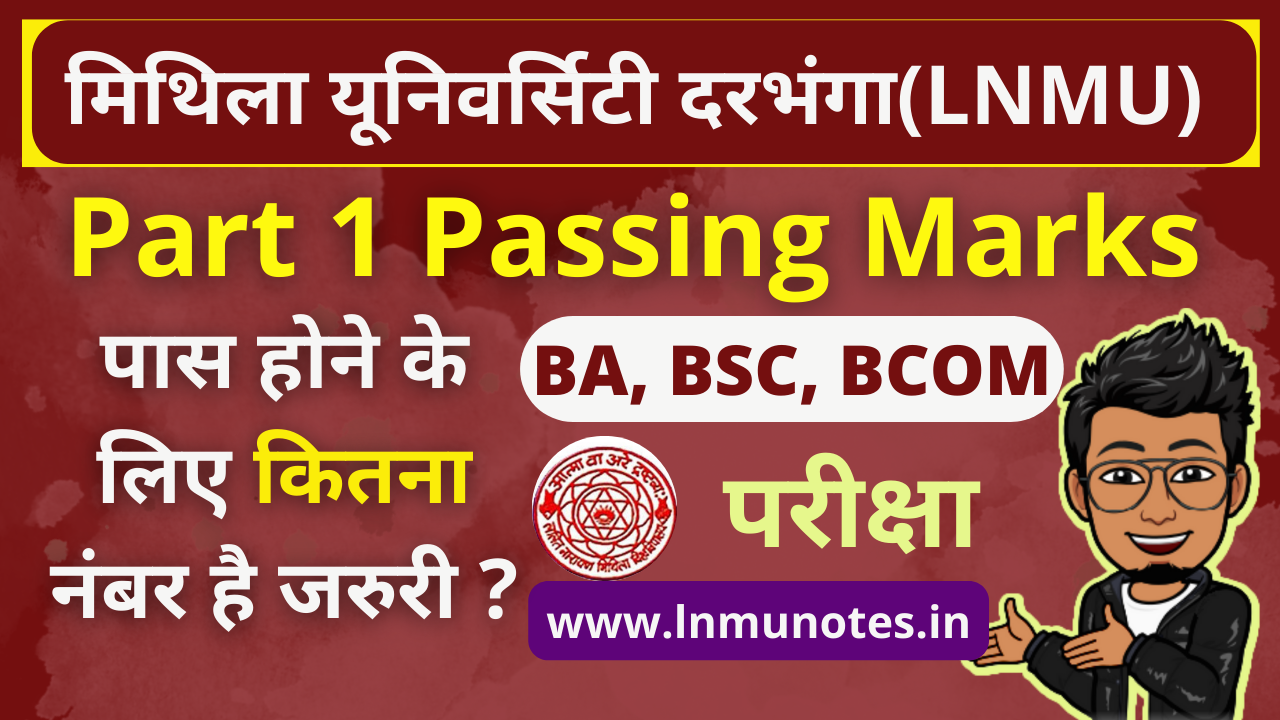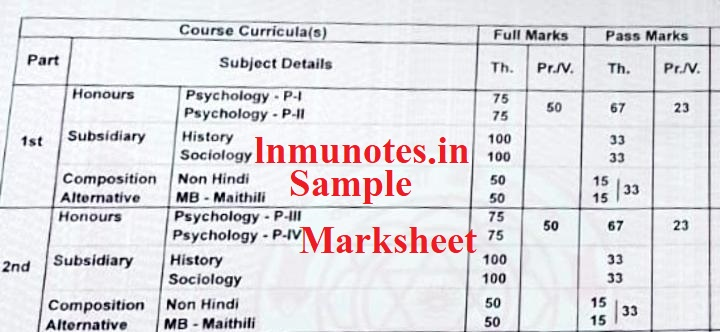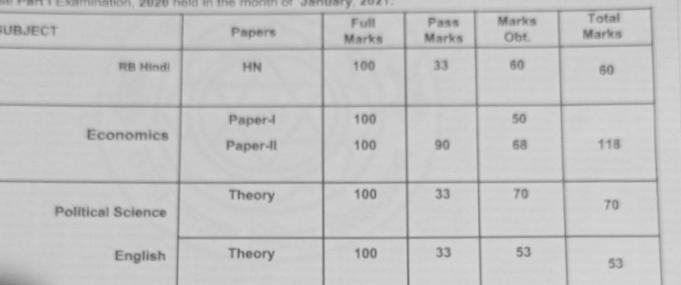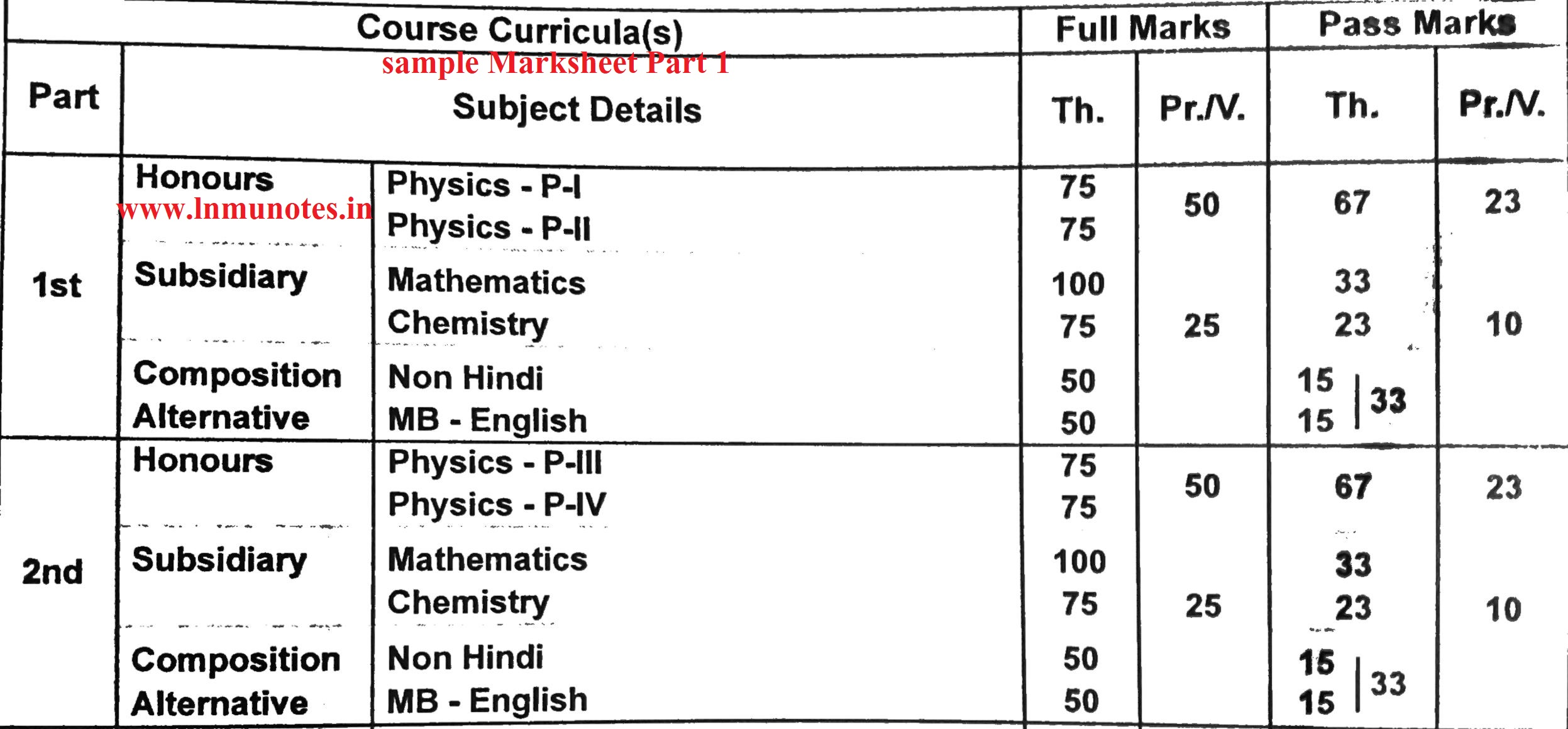# LNMU Part 1 Exam Passing Marks | परीक्षा पास होने के लिए कितना अंक जरुरी है BA, BSC, B.COM @lnmu.ac.in - lnmunotes.in

What is the minimum marks required to pass Part 1 exam in Lalit Narayan Mithila University Darbhanga? This post can be beneficial for the students of Arts, Commerce and Science of UG Part 1 or graduation first year, in this post the passing marks of almost all the subjects are given. This post can be read by part 1/first year students as well as part 2/second year students.

Highlights of this postLNMU Part 1 exam passing Marks

 Article LNMU Part 1 Exam Passing Marks Category Exam University Lalit Narayan Mithila University (LNMU) Course BA, BSC, B.Com Highlights Part 1 exam Total Marks Part 1 exam Pass Marks Part 1 exam Marksheet Sample LNMU Official Website lnmu.ac.in

"स्नातक प्रथम खण्ड के परीक्षा में पास होने के लिए कितने नंबर लाना जरुरी होता है , इसी सवाल का जवाब हम इस पोस्ट के माध्यम से देने की कोसिस करेंगे . मिथिला यूनिवर्सिटी के छात्रों के लिए "

# Lalit Narayan Mithila University Darbhanga Part 1 or First Year Graduation Course

Hi, you have also taken admission to complete your graduation from LNMU Darbhanga and are confused about the course then this post is for you. After taking admission in Part 1 new students are a little confused that how much they have to study for the exam. What is the syllabus of our subject, unless we see the syllabus, we cannot get an idea of ​​the pattern of questions in any exam and can not prepare for the exam properly.

After seeing the syllabus, we understand how much we have to study for the exam, but sometimes we also want to know how many marks we have to get to pass.

There are a total of 37 subjects for LNMU's 3-year degree course i.e. graduation. The list of all subjects are given below, if a student wants to do graduation in the given subjects, then it is mandatory for him/her to pass the Class 12th exam with 45% marks. However, if a student wants to change his/her stream i.e. Science / Commerce students want to take the subject of Arts, then it is mandatory for them to get 50% marks in 12th.

You can get an idea of ​​the Mithila University Part 1 Exam Pattern by looking at the previous years' questions.

#### LNMU Part 1 Exam Pattern

For the last 2 years i.e. from 2020, the university has made changes in the exam pattern, that is: All the honors subject examinations will be in the subjective mode and the examination of the subsidiary subjects will be taken in the objective mode. Whereas before 2020, all the exams were conducted in the subjective mode.

We have tried to solve the doubts that new students have regarding syllabus and passing marks.
Hope you like it. ,

## All Subjects List under Mithila University Darbhanga for Graduation

1. AIH (Ancient Indian History) and Culture
2. Anthropology
3. B.Com (Account and Finance)
4. B.Com (Marketing)
5. B.Com (Human Resource Management)
6. Physics
7. Chemistry
8. Mathematics (Science)
9. Mathematics (Arts)
10. Zoology
11. Botany
12. English
13. Hindi
14. Geography
15. Home Science
16. History
17. Political Science
18. Psychology
19. Persian
20. Philosophy
21. Maithili
22. Economics
23. Urdu
24. Sociology
25. Rular Economics
26. Music
27. Dramatics
28. Sanskrit
29. L.S.W (Labour And Social Welfare)
30. Bhojpuri
31. Pali
32. Nepali
33. Arabic
34. Bangla
35. Library Science
36. Prakrit
37. Statistics

## LNMU UG Part 1 BA (Bachelor of Arts) Honours Subject Passing Marks

AIH (Anient Indian History) and Culture Paper - 1 = 100 Marks
Paper -2 = 100 Marks
Paper -1 = 45 Pass Marks
Paper -2 = 45 Pass Marks
ANTHROPOLOGY Not Available Not Available Not Available
ARABIC Not Available Not Available Not Available
BANGLA Not Available Not Available Not Available
BHOJPURI Not Available Not Available Not Available
DRAMATICS Not Available Not Available Not Available
ECONOMICS Paper - 1 = 100 Marks
Paper -2 = 100 Marks
Paper -1 = 45 Pass Marks
Paper -2 = 45 Pass Marks
ENGLISH Paper - 1 = 100 Marks
Paper -2 = 100 Marks
Paper -1 = 45 Pass Marks
Paper -2 = 45 Pass Marks
GEOGRAPHY Paper - 1 = 75 Marks
Paper -2 = 75 Marks
Practical - 50 Marks
Paper -1 = 34 Pass Marks
Paper -2 = 34 Pass Marks
Practical - 23 Pass Marks
HINDI Paper - 1 = 100 Marks
Paper -2 = 100 Marks
Paper -1 = 45 Pass Marks
Paper -2 = 45 Pass Marks
HISTORY Paper - 1 = 100 Marks
Paper -2 = 100 Marks
Paper -1 = 45 Pass Marks
Paper -2 = 45 Pass Marks
HOME SCIENCE Paper - 1 = 75 Marks
Paper -2 = 75 Marks
Practical - 50 Marks
Paper -1 = 34 Pass Marks
Paper -2 = 34 Pass Marks
Practical - 23 Pass Marks
L.S.W. (LABOUR SOCIAL WELFARE) Not Available Not Available Not Available
LIBRARY SCIENCE Not Available Not Available Not Available
MAITHILI Paper - 1 = 100 Marks
Paper -2 = 100 Marks
Paper -1 = 45 Pass Marks
Paper -2 = 45 Pass Marks
MATHEMATICS (Arts) Paper - 1 = 90 Marks
Paper -2 = 90 Marks
Paper -1 = 28 Pass Marks
Paper -2 = 28 Pass Marks
MUSIC Paper - 1 = 100 Marks
Paper -2(Practical) = 100 Marks
Paper -1 = 45 Pass Marks
Paper -2 = 45 Pass Marks (Not Sure)
NEPALI Not Available Not Available Not Available
PALI Not Available Not Available Not Available
PERSIAN Not Available Not Available Not Available
PHILOSOPHY Paper - 1 = 100 Marks
Paper -2 = 100 Marks
Paper -1 = 45 Pass Marks
Paper -2 = 45 Pass Marks
POLITICAL SCIENCE Paper - 1 = 100 Marks
Paper -2 = 100 Marks
Paper -1 = 45 Pass Marks
Paper -2 = 45 Pass Marks
PRAKRIT Not Available Not Available Not Available
PSYCHOLOGY Paper - 1 = 75 Marks
Paper -2 = 75 Marks
Practical - 50 Marks
Paper -1 = 34 Pass Marks
Paper -2 = 34 Pass Marks
Practical - 23 Pass Marks
RURAL ECONOMICS Not Available Not Available Not Available
SANSKRIT Paper - 1 = 100 Marks
Paper -2 = 100 Marks
SOCIOLOGY Paper - 1 = 100 Marks
Paper -2 = 100 Marks
Paper -1 = 45 Pass Marks
Paper -2 = 45 Pass Marks
STATISTICS Not Available Not Available Not Available
URDU Paper - 1 = 100 Marks
Paper -2 = 100 Marks
Paper -1 = 45 Pass Marks
Paper -2 = 45 Pass Marks

### Sample Part 1 Marksheet (BA)LNMU BA Part 1 Marksheet Sample of Subject with PracticalLNMU BA Part 1 Marksheet Sample of Without Practical Subect

### UG Part 1 BSC (Bachelor of Science) Honours Subject Passing Marks

PHYSICS Paper - 1 = 75 Marks
Paper -2 = 75 Marks
Practical - 50 Marks
Paper -1 = 34 Pass Marks
Paper -2 = 34 Pass Marks
Practical - 23 Pass Marks
CHEMISTRY Paper - 1 = 75 Marks
Paper -2 = 75 Marks
Practical - 50 Marks
Paper -1 = 34 Pass Marks
Paper -2 = 34 Pass Marks
Practical - 23 Pass Marks
MATHEMATICS (SCIENCE) Paper - 1 = 90 Marks
Paper -2 = 90 Marks
Paper -1 = 28 Pass Marks
Paper -2 = 28 Pass Marks
ZOOLOGY Paper - 1 = 75 Marks
Paper -2 = 75 Marks
Practical - 50 Marks
Paper -1 = 34 Pass Marks
Paper -2 = 34 Pass Marks
Practical - 23 Pass Marks
BOTANY Paper - 1 = 75 Marks
Paper -2 = 75 Marks
Practical - 50 Marks
Paper -1 = 34 Pass Marks
Paper -2 = 34 Pass Marks
Practical - 23 Pass Marks

### Part 1 BSc Sample MARKSHEETLNMU BSC Part 1 Marksheet Sample

### UG Part 1 B.Com (Bachelor of Commerce) Honours Subject Passing Marks

ACCOUNTING AND FINANCE Paper - 1 = 100 Marks
Paper -2 = 100 Marks
Paper -1 = 45 Pass Marks
Paper -2 = 45 Pass Marks
HUMAN RESOURCE MANAGEMENT Paper - 1 = 100 Marks
Paper -2 = 100 Marks
Paper -1 = 45 Pass Marks
Paper -2 = 45 Pass Marks
MARKETING Paper - 1 = 100 Marks
Paper -2 = 100 Marks
Paper -1 = 45 Pass Marks
Paper -2 = 45 Pass Marks

### UG Part 1 Subsidiary Subject Passing Marks

PHYSICS Full Marks (Theory) = 75 Marks
Full Marks (Practical) = 25 Marks
Theory = 23 Pass Marks
Practical = 10 Pass Marks
CHEMISTRY Full Marks (Theory) = 75 Marks
Full Marks (Practical) = 25 Marks
Theory = 23 Pass Marks
Practical = 10 Pass Marks
ZOOLOGY Full Marks (Theory) = 75 Marks
Full Marks (Practical) = 25 Marks
Theory = 23 Pass Marks
Practical = 10 Pass Marks
BOTANY Full Marks (Theory) = 75 Marks
Full Marks (Practical) = 25 Marks
Theory = 23 Pass Marks
Practical = 10 Pass Marks
PSYCHOLOGY Full Marks (Theory) = 75 Marks
Full Marks (Practical) = 25 Marks
Theory = 23 Pass Marks
Practical = 10 Pass Marks
HOME SCIENCE Full Marks (Theory) = 75 Marks
Full Marks (Practical) = 25 Marks
Theory = 23 Pass Marks
Practical = 10 Pass Marks
GEOGRAPHY Full Marks (Theory) = 75 Marks
Full Marks (Practical) = 25 Marks
Theory = 23 Pass Marks
Practical = 10 Pass Marks
Other Subjects Full Marks = The Subjects in which Parctical exam is taken, the theory paper exam will be of 75 Marks and Practical exam of 25 marks
The Subjects in which Parctical exam is not taken, the theory paper exam will be of 100 Marks
If there is a Subsidiary Paper of 75 marks then there will be 23 pass marks and in Practical exam of 25 Marks there will be 10 passing marks
In 100 Marks of Subsidiary Paper there will be 33 passing Marks

#### Our Conclusion

Summarizing this post and adding some of your points, then the things that come out are:
1. 1. Part 1 exam pattern LNMU Darbhanga
• Honors subject exam will be subjective
• Subsidiary subject exams will be objective
1. 2. Part 1 passing marks LNMU Darbhanga
• If there is a practical in the subject with honours, then there will be 75 marks in a paper, in which you will have to get 34 marks to pass.(If the marks of Honors Paper 1 and Paper 2 together is 67 marks then you have passed in Honors paper.)
• Practical will be of 50 marks (in Honours Subject) in which you have to score 23 marks
•  In those Honours subjects which do not have practical, you have to get 45 marks out of 100 marks paper to pass in that subject.
Subsidiary Subject :
• In Subsidiary Subjects which have practical, you have to score 23 marks out of 75 marks and 10 marks out of 25 marks in practical to pass
• You have to score 33 out of 100 in the subsidiary subject without practical
This is a kind of frequently asked questions asked by the students of Part 1 which we have tried to answer, hope you like it. You can send us your thoughts on the link given below.